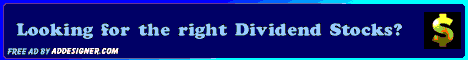Dividends4Life: 7 High-Quality Dividend Stocks To Buy

## 7 High-Quality Dividend Stocks To Buy

Posted by D4L | Tuesday, July 10, 2018 | | 0 comments »

Long-term investors know that dividends can have a major impact on returns over time. When the stock market is struggling to make gains like it has in 2018, dividends are a reliable source of income. However, dividends are only beneficial if the underlying stock is a high-quality stock. Bank of America Corp. (ticker: BAC) recently screened to find the highest-quality dividend stocks in its coverage universe. The analyst team identified following seven stocks as the only ones with a quality grade of "A" and a dividend yield at or above the S&P 500's average of 1.8 percent...

Automatic Data Processing (ADP) has a dividend yield of 1.8 percent, a free-cash-flow-to-dividend ratio of 1.8, a return on equity of 43.7 percent and a debt-equity ratio of just 0.5 percent. C.H. Robinson Worldwide (CHRW) has a dividend yield of 2.1 percent, a FCF-dividend ratio of 2.3, a ROE of 37.5 and a debt-equity ratio of 0.9. Emerson Electric Co. (EMR) has a dividend yield of 2.7 percent, a FCF-dividend ratio of 1.7, a ROE of 21.5 percent and a debt-equity ratio of 0.6. Genuine Parts Co. (GPC) has a dividend yield of 3 percent, a FCF-dividend ratio of 1.6, a ROE of 18.8 percent and a debt-equity ratio of 0.9. NextEra Energy (NEE) has a dividend yield of 2.4 percent, a FCF-dividend ratio of 3.3, a ROE of 28.3 percent and a debt-equity ratio of 1. Procter & Gamble Co. (PG) has a dividend yield of 3.8 percent, a FCF-dividend ratio of 1.2, a ROE of 18.6 percent and a debt-equity ratio of 0.6. United Technologies Corp. (UTX) has a dividend yield of 2.2 percent, a FCF-dividend ratio of 2.1, a ROE of 15.4 percent and a debt-equity ratio of 0.9.

Source: Yahoo Finance

Related Articles:
- 5 Best U.S. Dividend Growth Stocks
- 2 Low P/E Value-Stocks, Yielding 4% Or Higher
- How Much Money Will You Need To Retire?
- Seeding A Forest Of Dividend Growth Stocks
- 4 Stocks With A Strong Cash To Dividend Coverage

________________________________________________________________